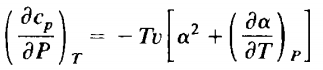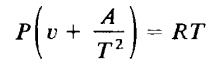### Create an Account

Home / Questions / One mole of the system of Problem 74 8 is expanded isothermally from an initial pressure P...

# One mole of the system of Problem 74 8 is expanded isothermally from an initial pressure P0 to a final pressure P1 Calculate the heat flux to the system in this process Problem

One mole of the system of Problem 7.4-8 is expanded isothermally from an initial pressure P0 to a final pressure P1. Calculate the heat flux to the system in this process.

Problem 7.4-8

Show thatEvaluate this quantity for a system obeying the equation of stateJun 09 2020 View more View LessSubscribe To Get Solution